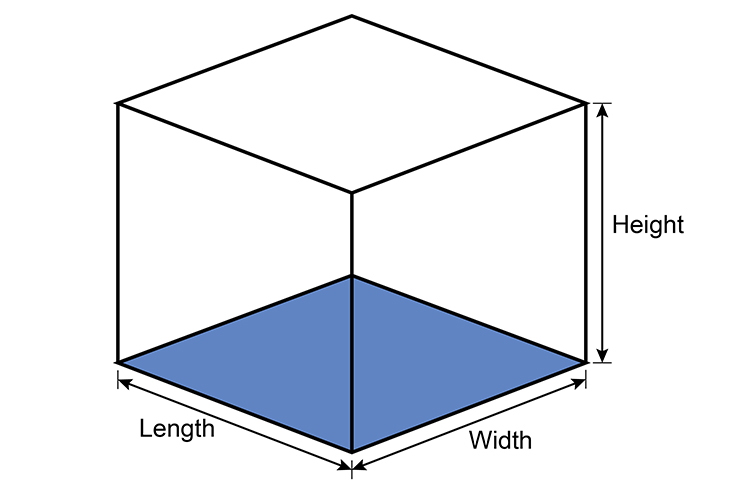# Volume of cubes

The volume of a cube works on area of the base multiplied by height and some factor.Volume of a cube = Area of base x Height

Area of base = Length x Width

Therefore

Volume of a cube = Length x Width x Height